library(tidyverse)
library(targets)
library(scales)
library(patchwork)
library(kableExtra)
library(sf)
library(here)

withr::with_dir(here::here(), {

df_country_aid_laws <- filter(df_country_aid, laws)

mutate(summary_order = 1:n())

})

my_seed <- 1234
set.seed(my_seed)

# Overall data summary

num_countries <- df_country_aid_laws %>% distinct(gwcode) %>% nrow()
num_years <- df_country_aid_laws %>% distinct(year) %>% nrow()
year_first <- df_country_aid_laws %>% distinct(year) %>% min()
year_last <- df_country_aid_laws %>% distinct(year) %>% max()

Our data includes information about 142 countries across 24 years (from 1990–2013)

# Summary of variables in model

(From our AJPS submission): The values here are slightly different from what we had at ISA and MPSA (and our ISQ submission) because we’re now using V-Dem 8.0 and AidData 3.1.

And now the values are even different-er, since we’re using V-Dem 10.0 and have really clean data now.

vars_summarized <- df_country_aid_laws %>%
select(one_of(vars_to_summarize$term)) %>% mutate(total_oda = total_oda / 1000000) %>% mutate(gdpcap_log = exp(gdpcap_log)) %>% pivot_longer(names_to = "term", values_to = "value", everything()) %>% filter(!is.na(value)) %>% group_by(term) %>% summarize(N = n(), Mean = mean(value), Median = median(value), Std. Dev. = sd(value), Min = min(value), Max = max(value)) %>% ungroup() %>% left_join(vars_to_summarize, by = "term") %>% arrange(summary_order) %>% mutate(across(c(Mean, Std. Dev., Median, Min, Max), ~case_when( format == "percent" ~ percent_format(accuracy = 0.1)(.), format == "dollar" ~ dollar_format(accuracy = 1)(.), format == "number" ~ comma_format(accuracy = 0.1)(.)))) %>% mutate(N = comma(N)) %>% select(category, subcategory, Variable = term_clean_table, Source = source, Mean, Std. Dev., Median, Min, Max, N) vars_summarized %>% select(-category,   = subcategory) %>% kbl() %>% kable_styling() %>% pack_rows(index = table(fct_inorder(vars_summarized$category))) %>%
collapse_rows(columns = 1, valign = "top")
Variable Source Mean Std. Dev. Median Min Max N
Outcome
Total aid (constant 2011 USD, millions) OECD and AidData $1,316$2,968 $483$0 $63,233 3,293 Proportion of contentious aid OECD and AidData 7.1% 10.5% 3.3% 0.0% 100.0% 3,293 Proportion of aid to domestic NGOs USAID 4.1% 13.8% 0.1% 0.0% 100.0% 3,293 Proportion of aid to foreign NGOs USAID 12.9% 20.1% 0.7% 0.0% 100.0% 3,293 Treatment Total legal barriers 1.5 1.9 1.0 0.0 9.5 3,293 Barriers to advocacy 0.3 0.5 0.0 0.0 2.0 3,293 Barriers to entry 0.8 0.9 1.0 0.0 3.0 3,293 Barriers to funding 0.4 0.9 0.0 0.0 4.5 3,293 Core civil society index 0.6 0.3 0.7 0.0 1.0 3,293 Confounders Human rights and politics Electoral democracy index (polyarchy) 0.4 0.2 0.4 0.0 0.9 3,293 Political corruption index 0.6 0.3 0.7 0.0 1.0 3,293 Rule of law index 0.5 0.3 0.4 0.0 1.0 3,293 Civil liberties index 0.6 0.2 0.7 0.0 1.0 3,293 Physical violence index 0.6 0.3 0.6 0.0 1.0 3,293 Private civil liberties index 0.6 0.3 0.7 0.0 1.0 3,293 Economics and development GDP per capita (constant 2011 USD) UN$6,359 $10,440$2,726 $92$82,410 3,293
Trade as % of GDP UN 78.1% 46.0% 69.2% 1.9% 441.6% 3,293
Educational equality 0.1 1.3 -0.1 -3.2 3.6 3,293
Health equality 0.1 1.4 -0.1 -3.3 3.5 3,293
Infant mortality rate (deaths per 1,000 births) 45.3 34.6 35.9 2.2 171.0 3,293
Unexpected shocks Internal conflict in last 5 years UCDP/PRIO 0.3 0.5 0.0 0.0 1.0 3,293
Natural disasters EM-DAT 2.1 3.5 1.0 0.0 43.0 3,293

# Aid stuff

## Overall OECD aid

total_oecd_aid <- df_country_aid_laws %>%
summarise(total = dollar(sum(total_oda))) %>%
pull(total)

Low $4,071,293,867,271 93.8% plot_aid_contentiousness <- df_donor %>% filter(year >= 1990) %>% group_by(year, purpose_contentiousness) %>% summarise(total = sum(oda)) %>% mutate(perc = total / sum(total)) %>% filter(purpose_contentiousness == "High") %>% mutate(fake_facet_title = "Contentious aid (all OECD)") fig_oecd_contention <- ggplot(plot_aid_contentiousness, aes(x = year, y = perc)) + geom_line(size = 0.5) + scale_y_continuous(labels = percent_format(accuracy = 1)) + labs(x = NULL, y = "Percent") + coord_cartesian(xlim = c(1990, 2015)) + theme_donors() + facet_wrap(vars(fake_facet_title)) fig_oecd_contention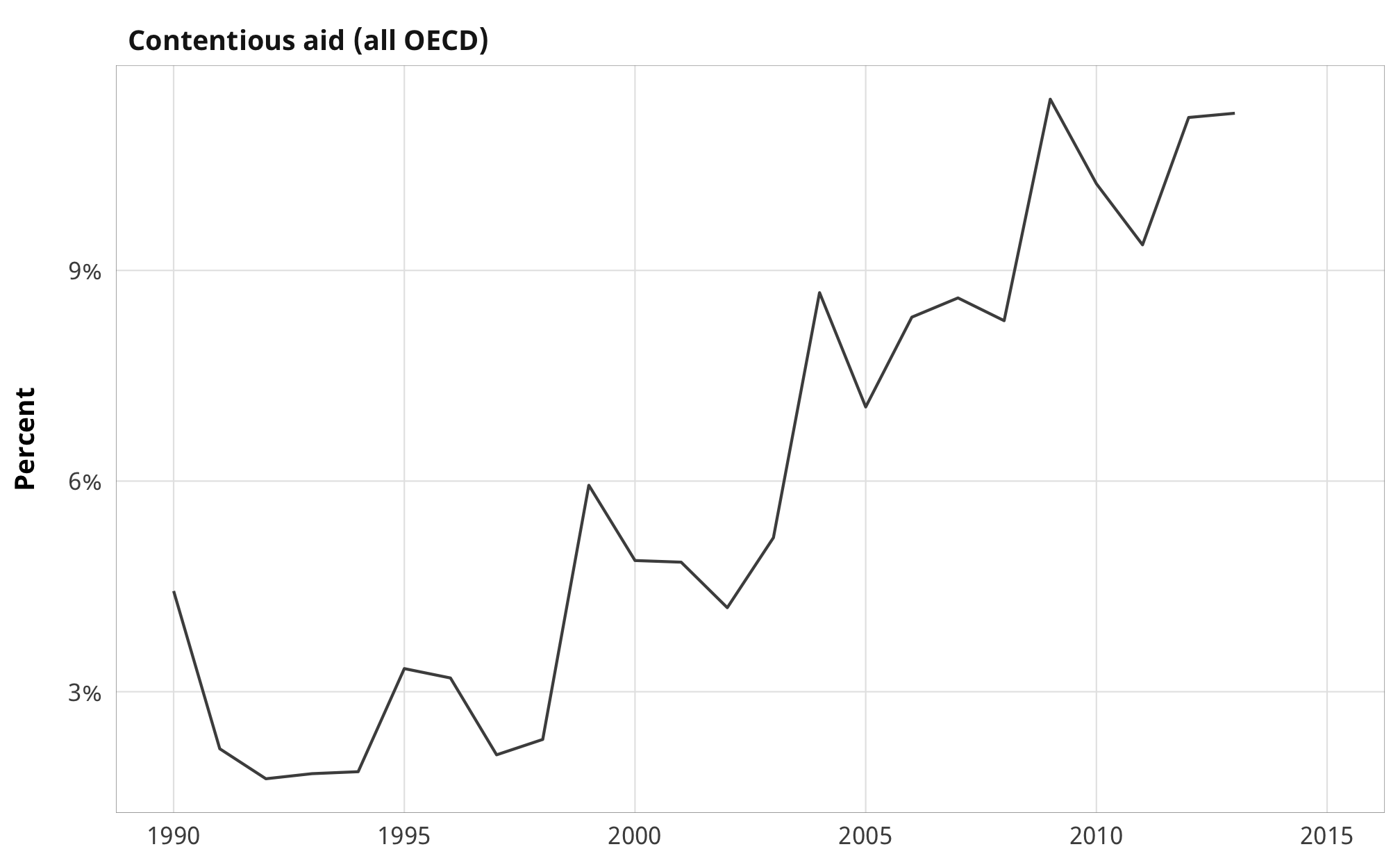## USAID aid total_us_aid <- df_country_aid_laws %>% summarise(total = dollar(sum(oda_us))) %>% pull(total) total_us_aid_post_2000 <- df_country_aid_laws %>% filter(year > 1999) %>% summarise(total = dollar(sum(oda_us))) %>% pull(total) The US donated$370,908,545,608 between 1990 and 2014 and $247,285,957,589 between 2000 and 2014. plot_aid_us <- df_country_aid_laws %>% filter(year >= 1990) %>% group_by(year) %>% summarise(total = sum(oda_us)) %>% mutate(fake_facet_title = "ODA commitments (USAID only)") fig_us_aid <- ggplot(plot_aid_us, aes(x = year, y = (total / 1000000000))) + geom_line(size = 0.5) + expand_limits(y = 0) + labs(x = NULL, y = "Billions of USD") + scale_y_continuous(labels = dollar) + coord_cartesian(xlim = c(1990, 2015)) + theme_donors() + facet_wrap(vars(fake_facet_title)) fig_us_aid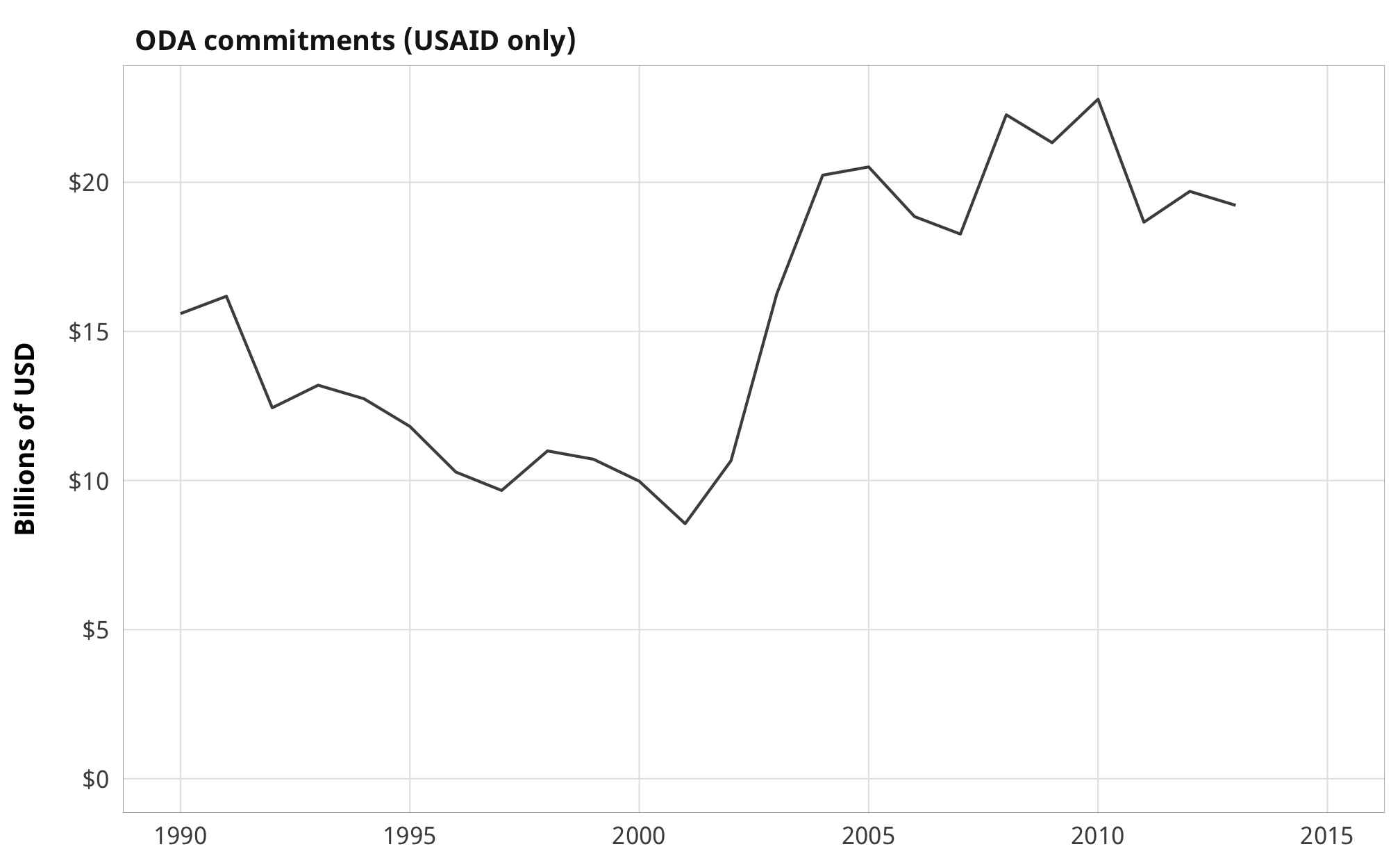## Proportion of US aid to types of NGOs Total amounts over time: usaid_total <- df_country_aid_laws %>% summarise(total = sum(oda_us)) %>% pull(total) channels_nice <- tribble( ~channel, ~channel_clean, "oda_us_ngo_dom", "Domestic NGOs", "oda_us_ngo_int", "International NGOs", "oda_us_ngo_us", "US-based NGOs" ) df_country_aid_laws %>% pivot_longer(names_to = "channel", values_to = "total_oda_us", c(oda_us_ngo_dom, oda_us_ngo_int, oda_us_ngo_us)) %>% group_by(channel) %>% summarise(total = sum(total_oda_us)) %>% ungroup() %>% left_join(channels_nice, by = "channel") %>% mutate(perc = total / usaid_total) %>% mutate(total = dollar(total), perc = percent(perc)) %>% select(Channel = channel_clean, Total = total, Percent = perc) %>% kbl() %>% kable_styling(full_width = FALSE) Channel Total Percent Domestic NGOs$6,244,919,475 1.7%
International NGOs $13,172,644,690 3.6% US-based NGOs$28,751,784,246 7.8%

The US clearly favors US-based NGOs or international NGOs over domestic NGOs.

usaid_total_yearly <- df_country_aid_laws %>%
group_by(year) %>%
summarise(annual_total = sum(oda_us)) %>%
mutate(fake_facet_title = "USAID ODA channeled through NGOs")

plot_usaid_channel <- df_country_aid_laws %>%
filter(year >= 1990) %>%
pivot_longer(names_to = "channel", values_to = "total_oda_us",
c(oda_us_ngo_dom, oda_us_ngo_int, oda_us_ngo_us)) %>%
group_by(year, channel) %>%
summarise(total = sum(total_oda_us)) %>%
left_join(usaid_total_yearly, by = "year") %>%
mutate(perc = total / annual_total) %>%
left_join(channels_nice, by = "channel")

fig_usaid_channel <- ggplot(plot_usaid_channel,
aes(x = year, y = perc, colour = channel_clean)) +
geom_line(size = 0.5) +
scale_y_continuous(labels = percent_format(accuracy = 1)) +
scale_colour_manual(values = channel_colors) +
labs(x = NULL, y = "Percent") +
guides(colour = guide_legend(title = NULL, reverse = TRUE, nrow = 2)) +
coord_cartesian(xlim = c(1990, 2015)) +
theme_donors() +
facet_wrap(vars(fake_facet_title))
fig_usaid_channel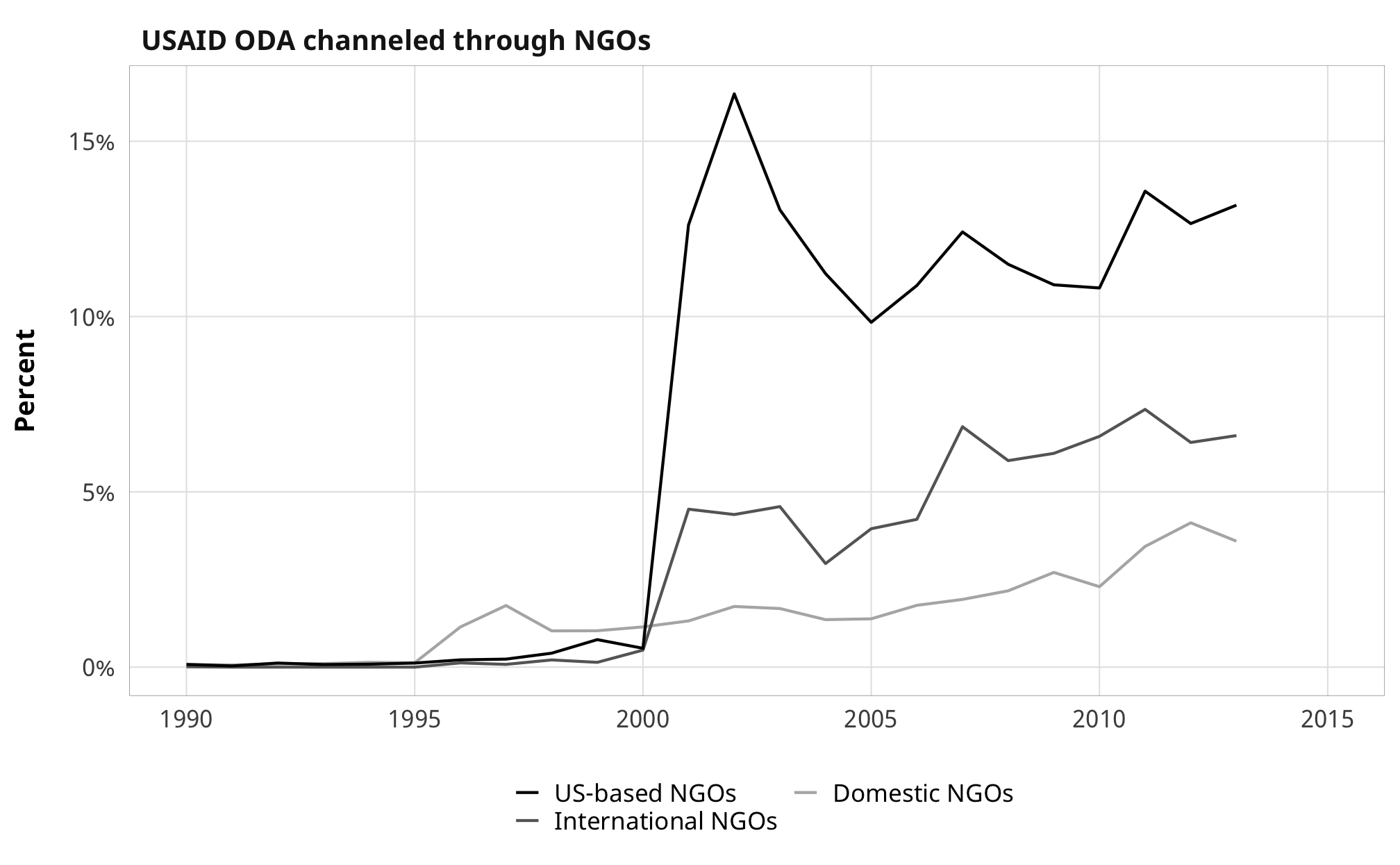USAID data categorizes all aid as government-channeled before 2000 because of some quirk in the data.

plot_usaid_channels_all <- df_donor_us %>%
group_by(year, channel_subcategory_name) %>%
summarise(total = sum(oda_us_2011)) %>%
mutate(perc = total / sum(total)) %>%
mutate(channel = ifelse(str_detect(channel_subcategory_name, "NGO"), "NGO", "Other"))

ggplot(plot_usaid_channels_all,
aes(x = year, y = perc, colour = channel_subcategory_name)) +
geom_line(size = 0.5) +
scale_y_continuous(labels = percent_format(accuracy = 1)) +
labs(x = NULL, y = "Percent of US aid given through different channels") +
guides(colour = guide_legend(title = NULL, ncol = 2)) +
theme_donors()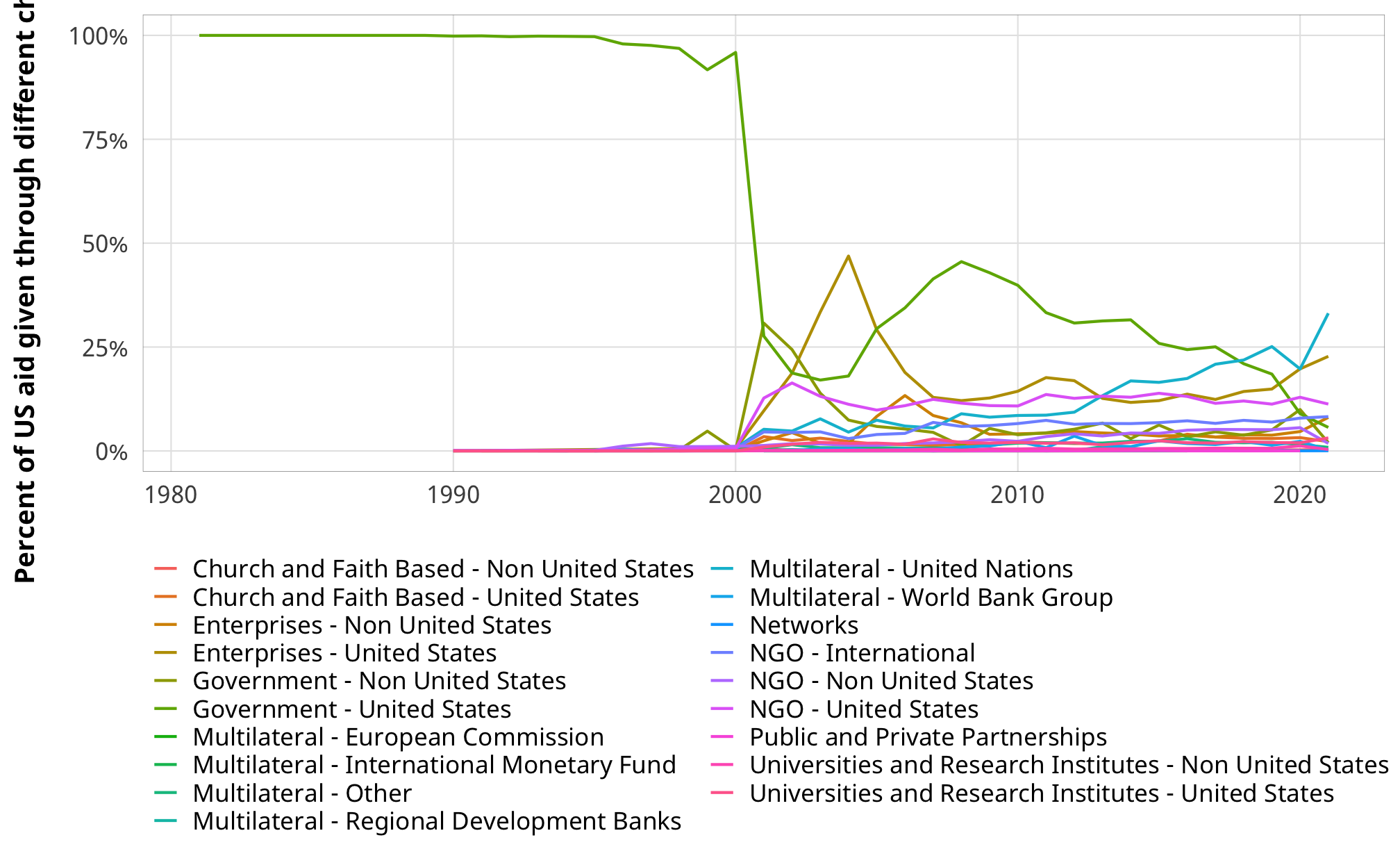So we just look at aid after 2000.

fig_usaid_channel_trimmed <- fig_usaid_channel +
coord_cartesian(xlim = c(2000, 2015))
fig_usaid_channel_trimmed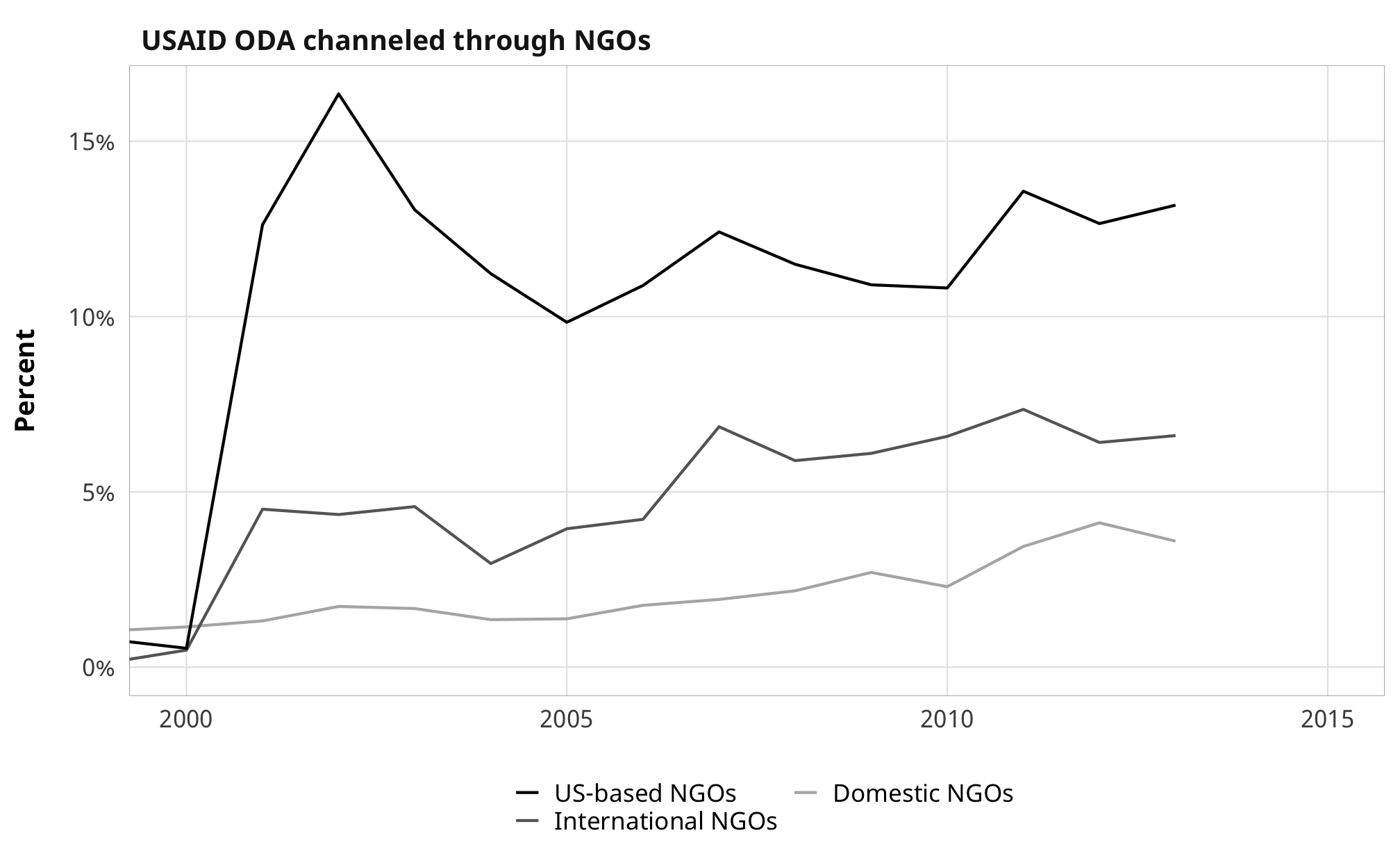## All DV figures combined

fig_dvs <- (fig_oecd_aid + fig_oecd_contention) /
(fig_us_aid + fig_usaid_channel_trimmed) &
theme_donors(base_size = 10) +
theme(legend.text = element_text(size = rel(0.6)),
axis.title.y = element_text(margin = margin(r = 3)),
legend.box.margin = margin(t = -0.5, unit = "lines"))

fig_dvs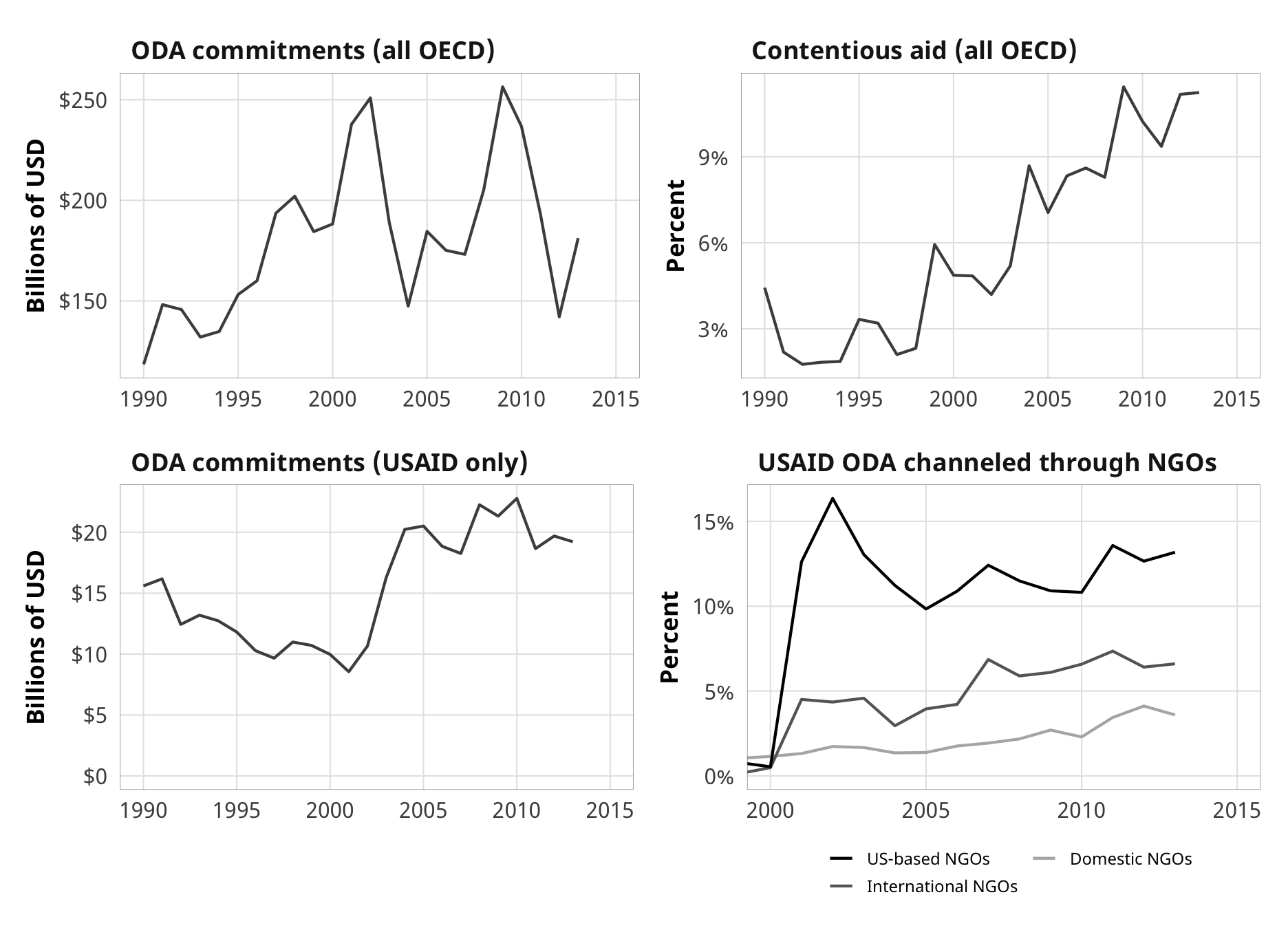ggsave(here("analysis", "output", "fig-dvs.pdf"), fig_dvs,
width = 6.5, height = 4.75, device = cairo_pdf)
ggsave(here("analysis", "output", "fig-dvs.png"), fig_dvs,
width = 6.5, height = 4.75, dpi = 300, type = "cairo")

# Aid

## Aid over time, by donor type

aid_donor_type_time <- df_donor %>%
group_by(year, donor_type_collapsed) %>%
summarise(total_aid = sum(oda, na.rm = TRUE))

ggplot(aid_donor_type_time, aes(x = year, y = total_aid / 1000000000,
colour = donor_type_collapsed)) +
geom_line(size = 0.5) +
labs(x = NULL, y = "Billions of USD",
caption = "Source: OECD and AidData. 2011 dollars.") +
guides(colour = guide_legend(title = NULL)) +
scale_y_continuous(labels = dollar) +
theme_donors()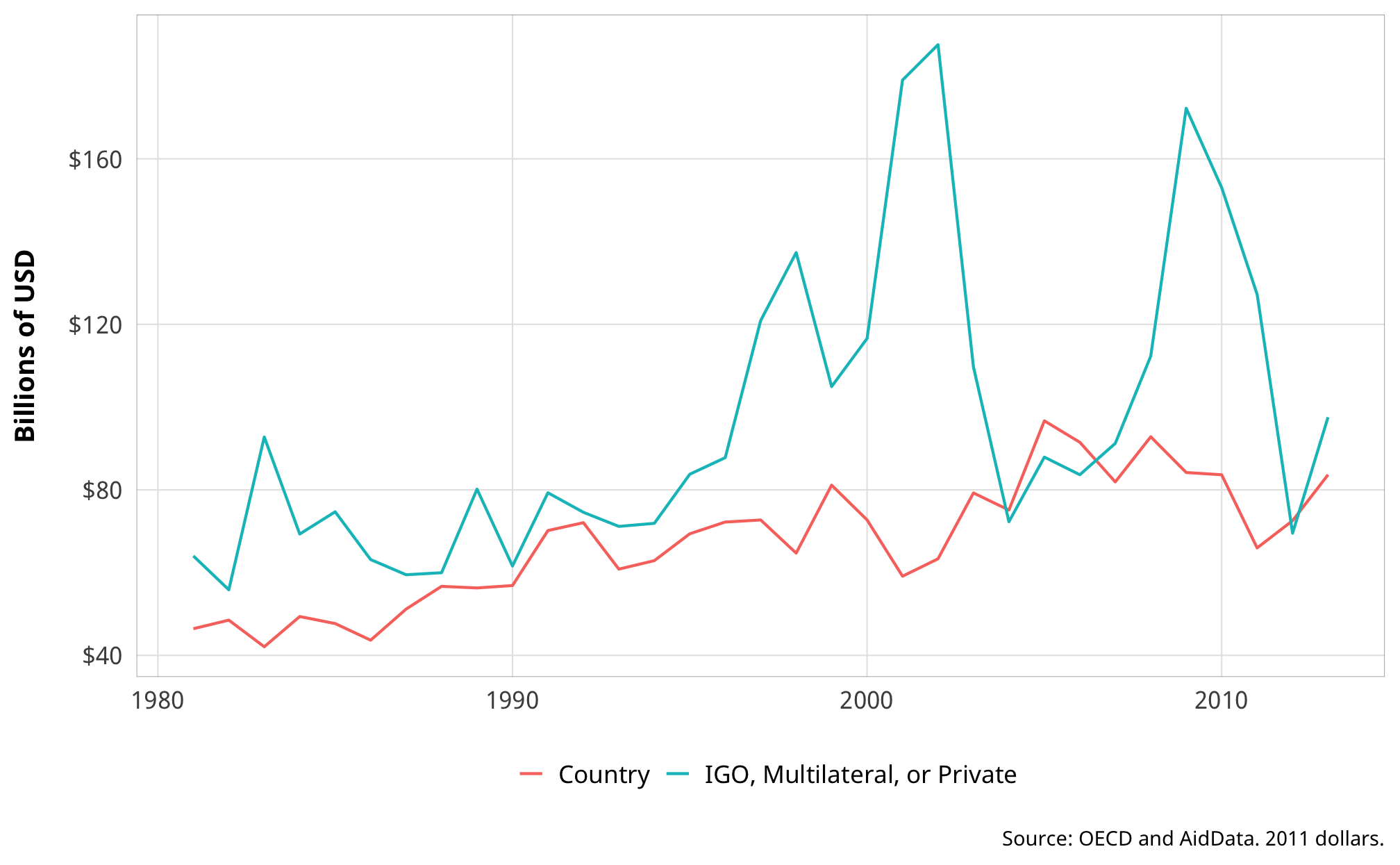## Aid over time, by contentiousness

aid_contention_time <- df_donor %>%
group_by(year, purpose_contentiousness) %>%
summarise(total_aid = sum(oda, na.rm = TRUE))

ggplot(aid_contention_time, aes(x = year, y = total_aid / 1000000000,
colour = purpose_contentiousness)) +
geom_line(size = 0.5) +
labs(x = NULL, y = "Billions of USD",
caption = "Source: OECD and AidData. 2011 dollars.") +
guides(colour = guide_legend(title = NULL)) +
scale_y_continuous(labels = dollar) +
theme_donors()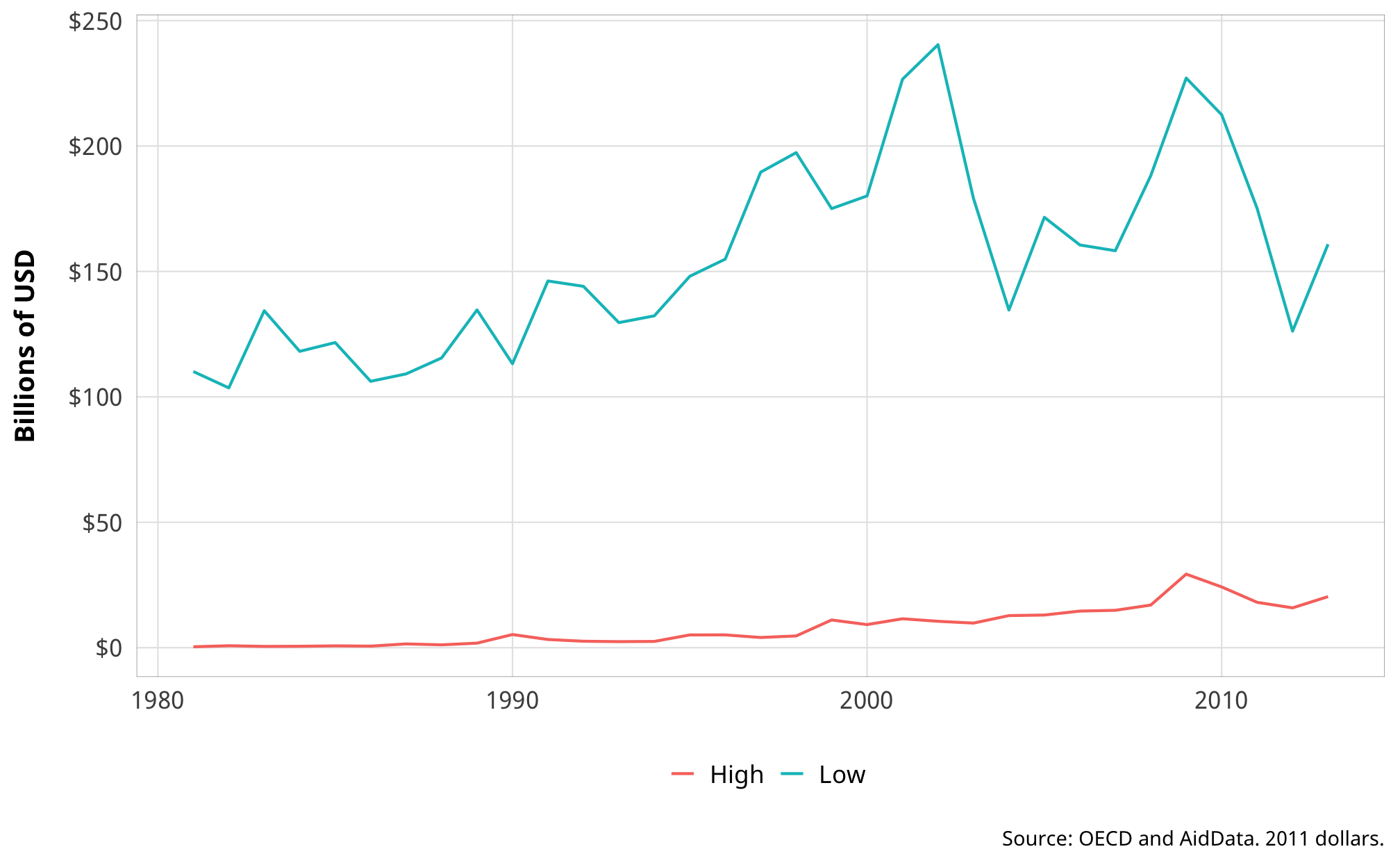# Restrictions and aid

inv_logit <- function(f, a) {
a <- (1 - 2 * a)
(a * (1 + exp(f)) + (exp(f) - 1)) / (2 * a * (1 + exp(f)))
}

dvs_clean_names <- tribble(
~barrier, ~barrier_clean,
"barriers_total", "All barriers",
"entry", "Barriers to entry",
"funding", "Barriers to funding"
)

ivs_clean_names <- tribble(
~variable, ~variable_clean, ~hypothesis,
"prop_ngo_dom_lead1", "Aid to domestic NGOs", "H3",
"prop_ngo_foreign_lead1", "Aid to foreign NGOs", "H3"
)

## Restrictions and ODA (H1)

df_plot_barriers_oda <- df_country_aid_laws %>%
one_of(dvs_clean_names$barrier)) %>% pivot_longer(names_to = "barrier", values_to = "value", one_of(dvs_clean_names$barrier)) %>%
left_join(dvs_clean_names, by = "barrier") %>%
mutate(barrier_clean = fct_inorder(barrier_clean, ordered = TRUE))

ggplot(df_plot_barriers_oda,
aes(x = value, y = total_oda_lead1, color = barrier_clean)) +
geom_point(alpha = 0.5) +
stat_smooth(method = "lm") +
stat_smooth(data = filter(df_plot_barriers_oda,
method = "lm", linetype = "21") +
scale_y_continuous(labels = dollar) +
guides(color = FALSE) +
labs(x = "Number of legal barriers", y = "Total ODA in next year",
title = "Total ODA in next year",
subtitle = "Dotted lines show trends when omitting observations\nwith less than $10,000,000,000 in ODA") + theme_donors(10) + theme(strip.text.x = element_text(margin = margin(t = 1, b = 1))) + facet_wrap(vars(barrier_clean), scales = "free_x", nrow = 2)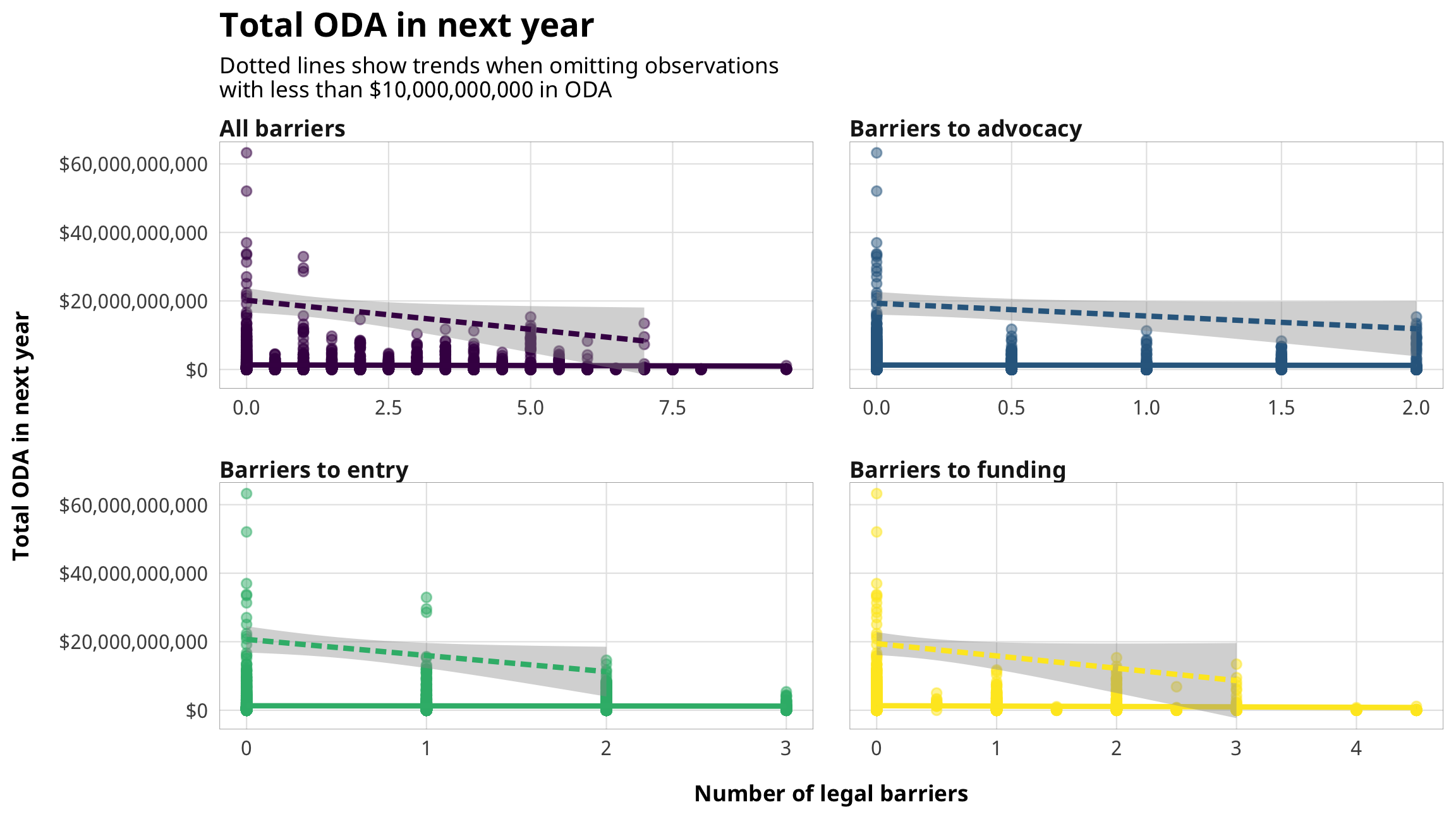## Restrictions and contentiousness (H2) df_plot_barriers_contention <- df_country_aid_laws %>% select(year, gwcode, country, prop_contentious_lead1, one_of(dvs_clean_names$barrier))  %>%
pivot_longer(names_to = "barrier", values_to = "value",
one_of(dvs_clean_names$barrier)) %>% left_join(dvs_clean_names, by = "barrier") %>% mutate(barrier_clean = fct_inorder(barrier_clean, ordered = TRUE)) ggplot(df_plot_barriers_contention, aes(x = value, y = prop_contentious_lead1, color = barrier_clean)) + geom_point(alpha = 0.5) + stat_smooth(method = "lm") + stat_smooth(data = filter(df_plot_barriers_contention, prop_contentious_lead1 > 0.05), method = "lm", linetype = "21") + scale_y_continuous(labels = percent) + guides(color = FALSE) + labs(x = "Number of legal barriers", y = "Proportion of contentious aid in next year", title = "Proportion of contentious aid in next year", subtitle = "Dotted lines show trends when omitting observations\nwith less than 5% contentious aid") + theme_donors(10) + theme(strip.text.x = element_text(margin = margin(t = 1, b = 1))) + facet_wrap(vars(barrier_clean), scales = "free_x", nrow = 2)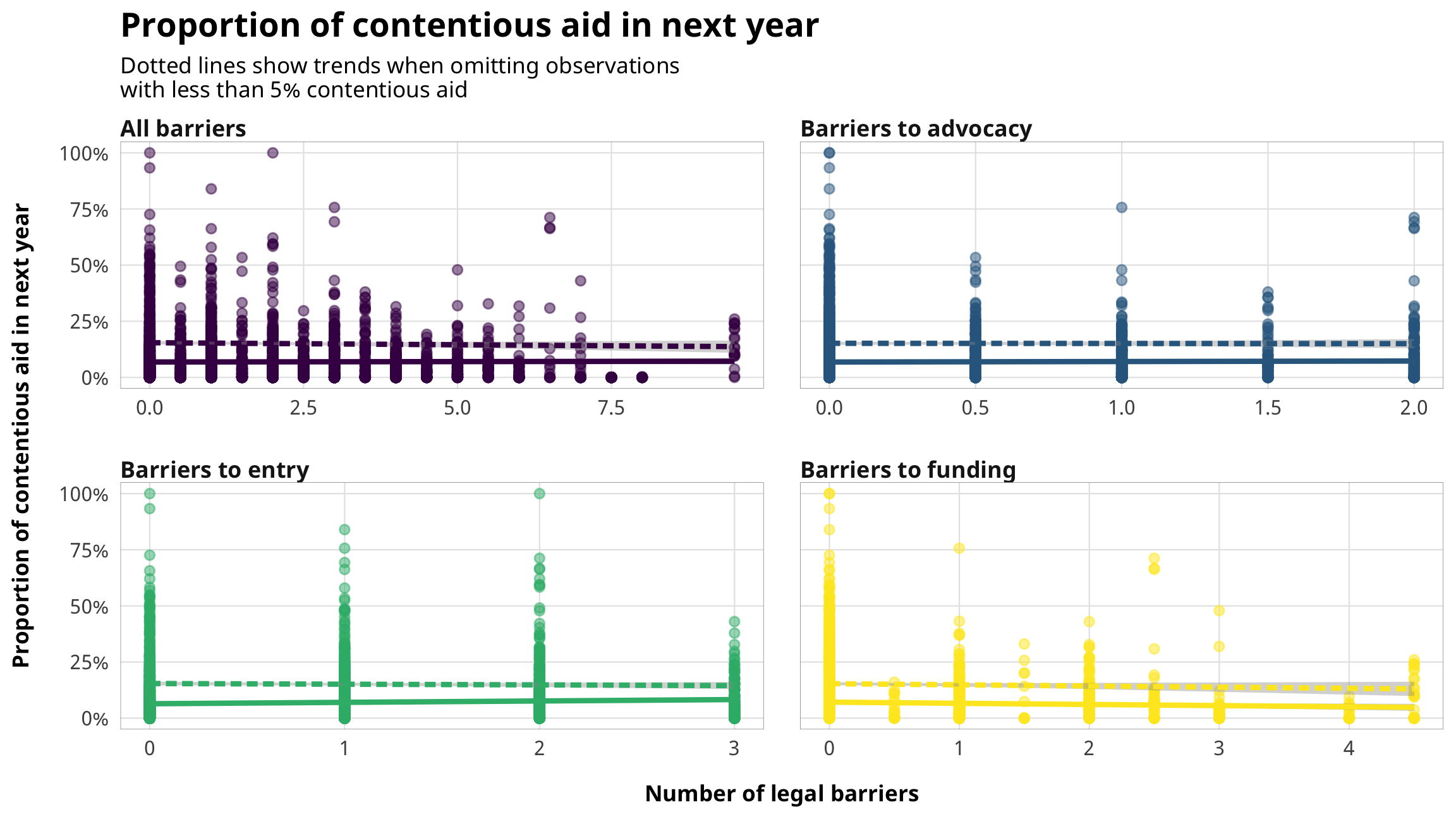## Restrictions and NGOs (H3) df_plot_barriers_ngos <- df_country_aid_laws %>% select(year, gwcode, country, prop_ngo_dom_lead1, prop_ngo_foreign_lead1, one_of(dvs_clean_names$barrier)) %>%
pivot_longer(names_to = "barrier", values_to = "value",
one_of(dvs_clean_names$barrier)) %>% pivot_longer(names_to = "ngo_type", values_to = "prop_ngo", c(prop_ngo_dom_lead1, prop_ngo_foreign_lead1)) %>% left_join(dvs_clean_names, by = "barrier") %>% left_join(ivs_clean_names, by = c("ngo_type" = "variable")) %>% mutate(barrier_clean = fct_inorder(barrier_clean, ordered = TRUE)) ggplot(df_plot_barriers_ngos, aes(x = value, y = prop_ngo, color = barrier_clean)) + geom_point(alpha = 0.5) + stat_smooth(method = "lm") + stat_smooth(data = filter(df_plot_barriers_ngos, prop_ngo > 0.05), method = "lm", linetype = "21") + scale_y_continuous(labels = percent) + guides(color = FALSE) + labs(x = "Number of legal barriers", y = "Proportion of aid to NGOs in next year", title = "Proportion of aid channeled to types of NGOs in next year", subtitle = "Dotted lines show trends when omitting observations\nwith less than 5% aid to NGOs") + coord_cartesian(ylim = c(0, 1)) + theme_donors(10) + theme(strip.text.x = element_text(margin = margin(t = 1, b = 1))) + facet_wrap(vars(variable_clean, barrier_clean), scales = "free_x", ncol = 4)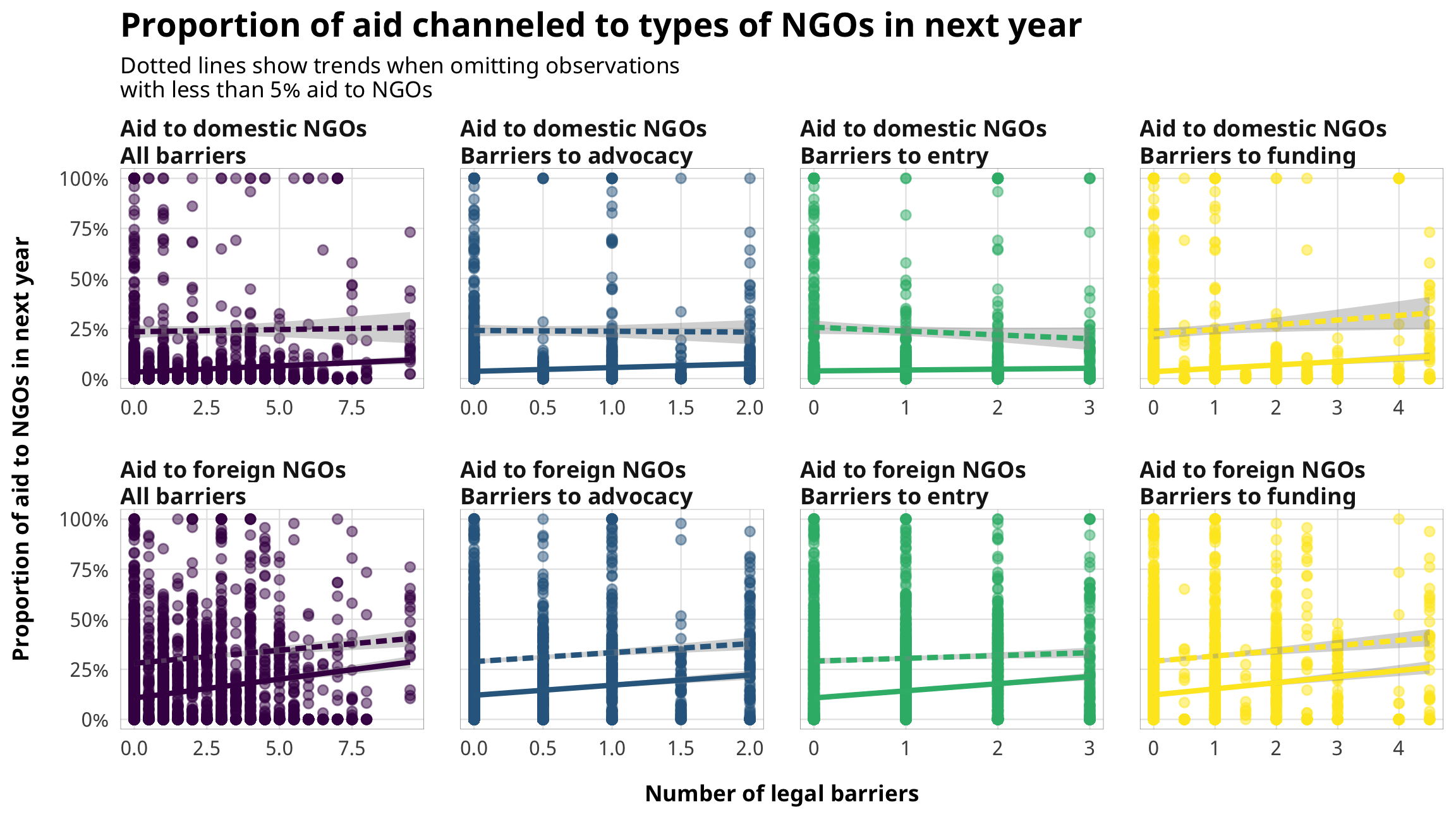## CCSI and all DVs (all hypotheses) df_plot_ccsi_ngos <- df_country_aid_laws %>% select(year, gwcode, country, one_of(ivs_clean_names$variable), v2xcs_ccsi) %>%
pivot_longer(names_to = "variable", values_to = "value",
c(one_of(ivs_clean_names\$variable))) %>%
left_join(ivs_clean_names, by = "variable") %>%
mutate(hypothesis_clean = paste0(hypothesis, ": ", variable_clean)) %>%
arrange(hypothesis_clean) %>%
mutate(hypothesis_clean = fct_inorder(hypothesis_clean, ordered = TRUE))

ggplot(df_plot_ccsi_ngos,
aes(x = v2xcs_ccsi, y = value, color = hypothesis)) +
geom_point(alpha = 0.25) +
scale_color_viridis_d(option = "plasma", end = 0.9) +
guides(color = FALSE) +
labs(x = "Civil society index",
y = "Variable value in next year",
title = "Core civil society index") +
theme_donors() +
facet_wrap(vars(hypothesis_clean), scales = "free_y")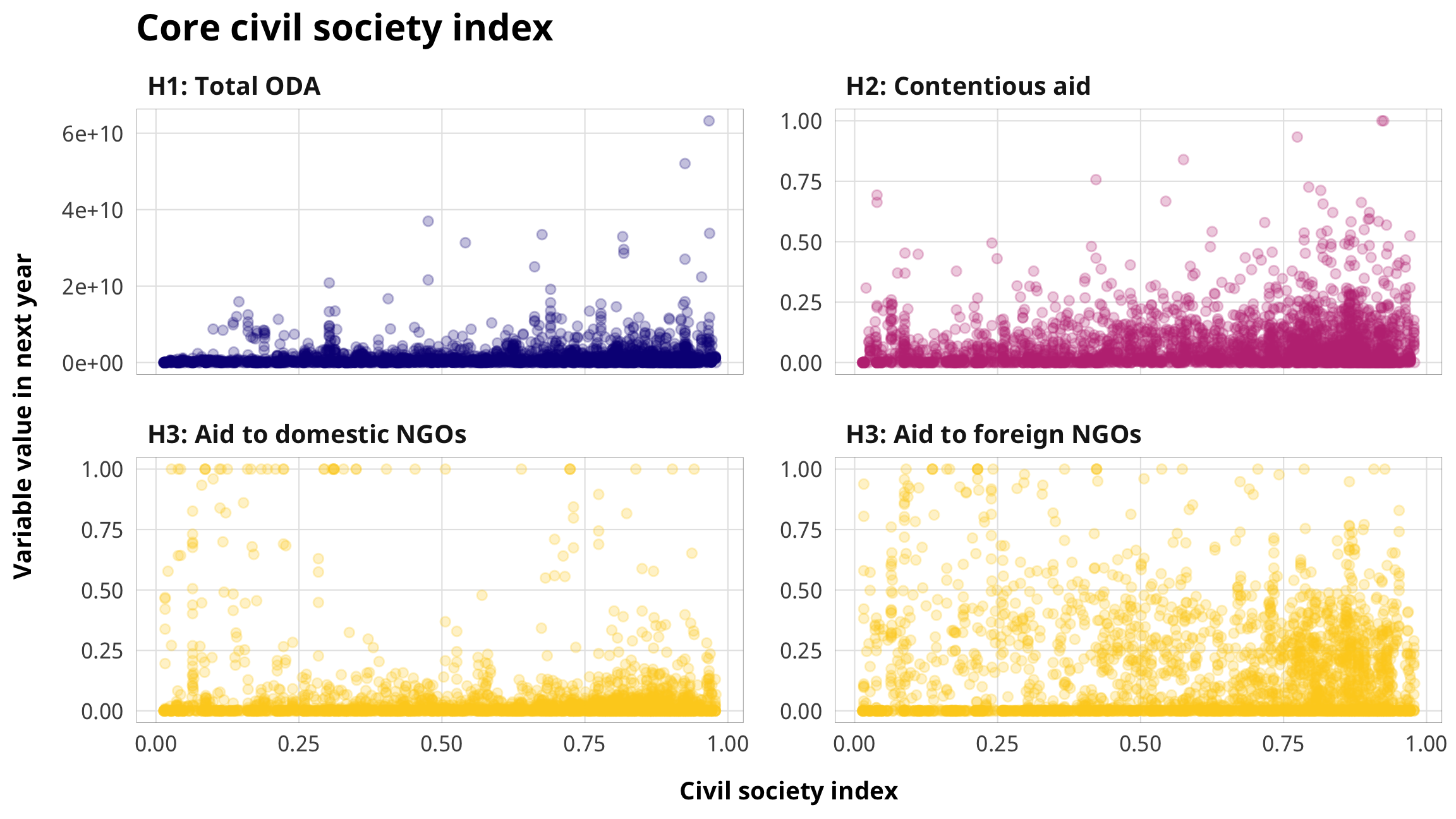# CIVICUS restrictions

civicus_clean %>%
filter(!is.na(category)) %>%
count(Rating = category, name = "Count") %>%
arrange(desc(Rating)) %>%
kbl(align = c("l", "c")) %>%
kable_styling(full_width = FALSE)
Rating Count
Open 42
Narrowed 40
Obstructed 47
Repressed 44
Closed 23
plot_civicus_map <- ggplot() +
geom_sf(data = civicus_map_data, aes(fill = fct_rev(category)), size = 0.15, color = "black") +
coord_sf(crs = st_crs("ESRI:54030"), datum = NA) +  # Robinson
# scale_fill_manual(values = c("grey90", "grey70", "grey45",
#                              "grey20", "black"),
#                   na.translate = FALSE, name = "Civic space") +
scale_fill_viridis_d(option = "plasma", end = 0.9,
na.translate = FALSE, name = "Civic space") +
theme_donors_map() +
theme(legend.position = "bottom",
legend.key.size = unit(0.7, "lines"))

plot_civicus_map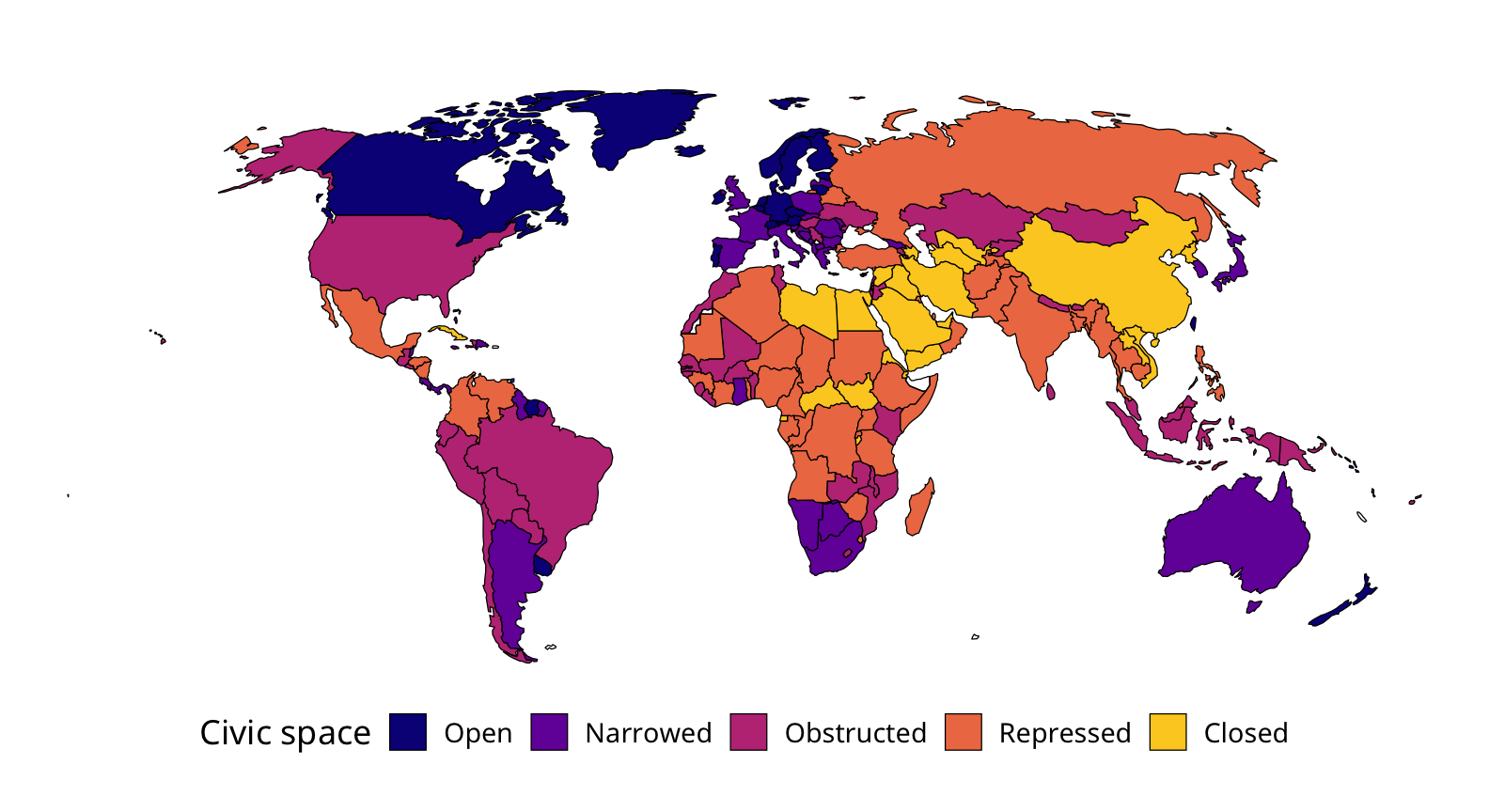ggsave(here("analysis", "output", "fig-civicus-map.pdf"), plot_civicus_map,
width = 5.5, height = 3, device = cairo_pdf)
ggsave(here("analysis", "output", "fig-civicus-map.png"), plot_civicus_map,
width = 5.5, height = 3, dpi = 300, type = "cairo")

# List of countries included in models

ncol_countries <- 4

all_countries <- df_country_aid_laws %>%
distinct(country) %>%
arrange(country) %>%
pull(country)

countries_caption <- paste0("All countries included in models (N = ",
length(all_countries), ")")

all_countries %>%
matrix_from_vector(ncol = ncol_countries) %>%
kbl(caption = countries_caption) %>%
kable_styling(bootstrap_options = c("condensed", "striped"),
full_width = FALSE)
 Afghanistan Ecuador Liberia Saudi Arabia Albania Egypt Libya Senegal Algeria El Salvador Lithuania Serbia Angola Equatorial Guinea Madagascar Sierra Leone Argentina Eritrea Malawi Singapore Armenia Estonia Malaysia Slovakia Azerbaijan Eswatini Mali Slovenia Bahrain Ethiopia Mauritania Solomon Islands Bangladesh Fiji Mauritius Somalia Belarus Gabon Mexico South Africa Benin Gambia Moldova South Korea Bhutan Georgia Mongolia South Sudan Bolivia Ghana Montenegro Sri Lanka Bosnia & Herzegovina Guatemala Morocco Sudan Botswana Guinea Mozambique Syria Brazil Guinea-Bissau Myanmar (Burma) Tajikistan Bulgaria Guyana Namibia Tanzania Burkina Faso Haiti Nepal Thailand Burundi Honduras Nicaragua Timor-Leste Cambodia Hungary Niger Togo Cameroon India Nigeria Trinidad & Tobago Central African Republic Indonesia North Korea Tunisia Chile Iran North Macedonia Turkey China Iraq Oman Turkmenistan Colombia Israel Pakistan Uganda Comoros Jamaica Panama Ukraine Congo - Brazzaville Jordan Papua New Guinea United Arab Emirates Congo - Kinshasa Kazakhstan Paraguay Uruguay Costa Rica Kenya Peru Uzbekistan Côte d’Ivoire Kosovo Philippines Venezuela Croatia Kuwait Poland Vietnam Cuba Kyrgyzstan Portugal Yemen Cyprus Laos Qatar Zambia Czechia Latvia Romania Zimbabwe Djibouti Lebanon Russia Dominican Republic Lesotho Rwanda